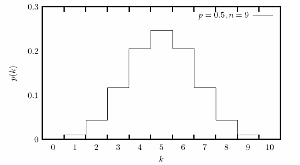Top Previous Next

# 4. Distributions of random values

A distribution function can be described by a density function: The probability that a specific value will occur. Non-uniform distributions are almost always computed starting with a uniform distribution. There are several different approaches. Sometimes one just applies a mathematical transformation. In other cases, more complex algorithms must be used.

Below are a few plots showing examples of non-uniform probability distributions often used in connection with RNGs.

### Uniform distribution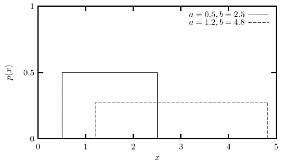### Normal (Gaussian) distribution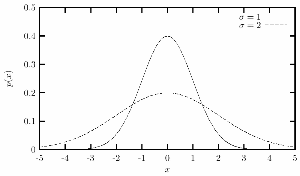### Exponential distribution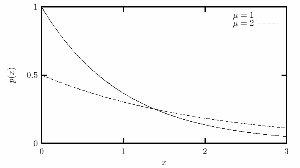### Gamma distribution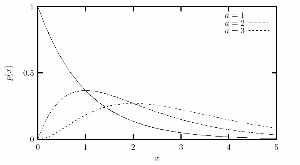### Poisson distribution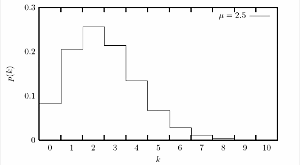### Binomial distribution